Courses

# Test: Inventory Control (Level - 1)

## 20 Questions MCQ Test Manufacturing and Industrial Engineering | Test: Inventory Control (Level - 1)

Description
This mock test of Test: Inventory Control (Level - 1) for Mechanical Engineering helps you for every Mechanical Engineering entrance exam. This contains 20 Multiple Choice Questions for Mechanical Engineering Test: Inventory Control (Level - 1) (mcq) to study with solutions a complete question bank. The solved questions answers in this Test: Inventory Control (Level - 1) quiz give you a good mix of easy questions and tough questions. Mechanical Engineering students definitely take this Test: Inventory Control (Level - 1) exercise for a better result in the exam. You can find other Test: Inventory Control (Level - 1) extra questions, long questions & short questions for Mechanical Engineering on EduRev as well by searching above.
QUESTION: 1

Solution:
QUESTION: 2

Solution:
QUESTION: 3

### In infinite replenishment model if the lead time increases from 20 to 30 days, the EOQ will

Solution: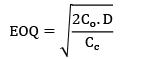QUESTION: 4

Consider the following inventory costs

1. cost of inspection

2. scrap cost

3. insurance cost

4. cost of negotiation with suppliers

Which of these costs are related to carrying cost?

Solution:
QUESTION: 5

Which of the following cost elements are considered while determining the Economic Lot Size for purchase in basic EOQ model?

1. Inventory carrying cost

2. Procurement cost

3. Set up cost

Select the correct answer using the codes given below Codes:

Solution:
QUESTION: 6

Which of the following statement is correct regarding EOQ?

Solution:
QUESTION: 7

In basic EOQ model, EOQ is dependent on which of the following?

1. ordering cost

2. unit cost

Solution:
QUESTION: 8

In fixed order quantity system i.e., Q-system, orders are placed whenever

Solution:
QUESTION: 9

The number of orders for a period is calculated using which of the following formula

Solution:
QUESTION: 10

At EOQ the total variable cost doesn’t depend on which of the following

Solution: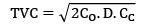QUESTION: 11

Formula of EOQ for a basic EOQ model doesn’t contain

Solution:
QUESTION: 12

Which one of the following is an inventory system that keeps a running record of the amount in storage and replenishes the stock when it drops to a certain level by ordering a fixed quantity?

Solution:
QUESTION: 13

ABC analysis is done to

Solution:
QUESTION: 14

Inventory classification based on criticality of usage is done in which of the following techniques

Solution:

VED

V-Vital

E-Essential

D-Desirable

QUESTION: 15

Which of the following selective inventory control technique is based on unit price of the items

Solution:

HML

H-High cost

M-Medium cost

L-Low cost

QUESTION: 16

In ABC analysis, A items require

Solution:
QUESTION: 17

Classifying items in A, B and C categories for selective control in inventory management is done by arranging items in the decreasing order of

Solution:
QUESTION: 18

In the ABC method of inventory control, Group A constitutes costly items. What is the usual percentage of such items of the total items?

Solution:
QUESTION: 19

In the EOQ model, if the unit ordering cost is doubled, the EOQ

Solution: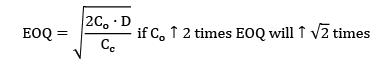*Answer can only contain numeric values
QUESTION: 20

The annual demand for a commodity is 100 units. The ordering cost per order is Rs. 800 and carrying cost is Rs. 0.16 per unit per year. Find the optimal ordering quantity.

Solution: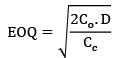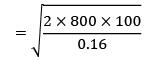EOQ = 1000 units